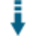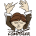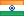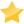# Profit and Loss PHP Calculator: Math class that can calculate profit and lossDownload .zip
 InfoExampleView files (4)Download .zip Reputation Support forum Blog (1) Links
 Last Updated Ratings Unique User Downloads Download Rankings 2023-01-27 (10 months ago)Not yet rated by the users Total: 61 All time: 10,357 This week: 204Version License PHP version Categories profitandlosscalcula 1.0 Free For Educatio... 5 PHP 5, Finances, Math, Global
Description

Author

This package provides a simple math class that can calculate profit and loss.

It provides a class that can perform addition and subtraction of amount values.

The package comes with an example script that presents a form for the user to enter the cost price and selling prices, and then it tells the user if there was a profit or loss.

Another example script can calculate the cost prices if it is missing from the selling price and the profit and loss percentages or can calculate the selling price if it is missing from the cost prices and the profit and loss percentages.

Innovation AwardJanuary 2023 Number 9 Finance is essential to measure the success or failure of a business in terms of whether it is earning or losing money. The package can help calculate the cost and selling prices of products or services for profit or loss percentages. Manuel LemosPerformance Level
Name: Classes: Dev Sharma `` 4 packages by Dev SharmaIndia 21 3207 209 in India39834 in IndiaLevel 1Innovation awardNominee: 2x

## Example

 ``` Profit and Loss - Simple calculator
"; echo 'Profit' . ' = ' . \$calc->sub(\$numOne, \$numTwo) . "\n"; // we will now minus the inputs as per the formula echo 'Loss' . ' = ' . \$calc->sub(\$numTwo, \$numOne) . "\n"; // we will now minus the inputs as per the formula } if(\$numOne > \$numTwo) { echo 'Therefore, as your cost price is higher than the selling price; there is a loss.'; echo "
"; echo ' Loss ' . ' = ' . "\n" . \$calc->sub(\$numOne, \$numTwo); // we will now minus the inputs as per the formula \$losspercent = \$calc->sub(\$numOne, \$numTwo); echo ' Loss Percentage ' . ' = ' . round((\$losspercent / \$numOne) * 100, 2) . '%'; //we will now find out the percentage; loss/total cost * 100 as per the formula and round up any decimal numbers to 2 decimal places. } if(\$numTwo > \$numOne) { echo 'Therefore, as your selling price is higher than the cost price; there is a profit.'; echo "
"; echo 'Profit' . ' = ' . "\n" . \$calc->sub(\$numTwo, \$numOne); // we will now minus the inputs as per the formula \$profitpercent = \$calc->sub(\$numTwo, \$numOne); echo ' Profit Percentage ' . ' = ' . round((\$profitpercent / \$numOne) * 100, 2) . '%'; //we will now find out the percentage; profit/total cost * 100 as per the formula and round up any decimal numbers 2 decimal places. } } ?> ```

# ProfitandLossCalculator

Profit and Loss calculator is a simple calculator which helps you find the profit or loss using the cost price and the selling price with percentage which rounds up-to 2 decimal places if needed. The calculator only follows the formula it is meant to follow in the profit and loss formulas.

The advanced version helps you to find out the cost price or selling price(if it's missing from your problem sum) with the help of profit or your loss given below in your problem sum.

Enjoy!Files
File Role DescriptionAdvancedPnL.php Aux. Auxiliary scriptclass.calc.php Class Class sourcePnL.php Example Example scriptREADME.md Doc. Documentation

 All time: 10,357 This week: 204About usAdvertise on this siteSite mapNewsletterStatisticsSite tipsPrivacy policyContact
For more information send a message to `info at phpclasses dot org`.